# Probability and statistics tools and demonstrations¶

By Max Kapur. Updated Jan. 27, 2020.

These notes are written at the level of the concepts they address. That is, I try not to reference concepts from later sections when solving problems from early in the text.

In :
import numpy as np
import pandas as pd
import sympy
import matplotlib.pyplot as plt
import matplotlib.cm as cm
from mpl_toolkits.mplot3d import Axes3D
from scipy.special import binom
from scipy.stats import chi2, gamma, norm, t, rankdata
from scipy.optimize import minimize
from math import factorial


## Independent and dependent probability¶

Reference: Robert V. Hogg et al., Probability and Statistical Inference 9e, section 1.4.

A homework problem from this section reads,

An urn contains 10 red and 10 white balls. The balls are drawn from the urn at random, one at a time. Find the probabilities that the fourth white ball is the fourth, fifth, sixth, or seventh ball drawn if the sampling is done

1. With replacement.
2. Without replacement.
3. In the World Series, the American League (red) and National League (white) teams play until one team wins four games. Do you think that the urn model presented in this exercise could be used to describe the probabilities of a 4-, 5-, 6-, or 7-game series? (Note that either “red” or “white” could win.) If your answer is yes, would you choose sampling with or without replacement in your model? (For your information, the numbers of 4-, 5-, 6-, and 7-game series, up to and including 2012, were 21, 24, 23, 36. This ignores games that ended in a tie, which occurred in 1907, 1912, and 1922. Also, it does not include the 1903 and 1919–1921 series, in which the winner had to take five out of nine games. The World Series was canceled in 1994.)

I will use Python to demonstrate how list comprehensions can cut down on the busy work otherwise required by this problem. (Take a look at the ratio of text to code in this section.)

First, let’s think about what it means for the fourth white ball to occur on the nth trial.

There is only one way for the fourth white ball to occur on the fourth trial: all of the preceding balls must have also been white. For a reason that will be clear in a moment, we can express this one possible arrangement as the binomial coefficient $1 = \binom{3}{3}$.

For the fourth white ball to occur on the fifth trial, there must have been exactly three white balls on trials one through four (any more, and the experiment has terminated; any fewer, and we’ll need to run more trials). There are $\binom{4}{3} = 4$ ways for that to happen:

$$\text{white, white, white, red}\\ \text{white, white, red, white} \\\text{white, red, white, white} \\\text{red, white, white, white}$$

Likewise, if we draw the fourth white ball on trial six, there are $\binom{5}{3}$ ways for there to have been exactly three white balls drawn in the preceding five trials, and so on.

The number of “ways” for the fourth white ball to occur on the nth trial is then $\binom{n-1}{3}$. Let’s store those values for $n = \{4,5,6,7\}$ in the variable ways. We will need these coefficients to answer both parts of the question.

In :
ways = np.array([binom(n-1,3) for n in range(4,8)])
ways

Out:
array([ 1.,  4., 10., 20.])

#### Part A¶

For the fourth white ball to occur on a certain trial, we need to stick exactly to the sequences enumerated above, then complete each sequence by drawing a white ball on the nth trial itself. In part A, we are sampling with replacement, so the probability of selecting a given ball on a given trial is always $10/20 = 1/2$. That means that each sequence of length n occurs with probability $\mathrm{P}(S_n) = (1/2)^n$. Let’s store these probabilities in another vector, psn.

Note that $S_n$ denotes the probability of a given particular sequence of length n occurring, not the (higher) probability of “any sequence containing four white outcomes, with the white outcome coming up last.”

In :
psn = np.array([0.5**n for n in range(4,8)])
psn

Out:
array([0.0625   , 0.03125  , 0.015625 , 0.0078125])

Now, let $A_n$ denote the event of drawing the fourth ball on the nth trial, or using the language above, “any sequence containing four white outcomes, with the white outcome coming up last.” Well, we already figured out the number of length-n sequences that fit this description when we created the ways variable. Multiplying those coefficients by the corresponding terms of psn will give us the answer to part A.

In :
ways * psn

Out:
array([0.0625 , 0.125  , 0.15625, 0.15625])

This is $\frac{1}{16}, \frac{1}{8}, \frac{5}{32}, \frac{5}{32}$, the answer given in the book.

However, another way to interpret the question would be to find the probability of the fourth white ball occurring on any one of the four trials mentioned. This is $\mathrm{P}(A_4 \cup A_5 \cup A_6 \cup A_7 )$. Since the $A_n$ are mutually exclusive (you cannot draw the fourth white ball on trial four and then again on trial five), we know:

\begin{align} \mathrm{P}(A_4 \cup A_5 \cup A_6 \cup A_7 ) &= \mathrm{P}(A_4) + \mathrm{P}(A_5) + \mathrm{P}(A_6) + \mathrm{P}(A_7) \\ &= \binom{3}{3}\left(\frac{1}{2}\right)^4 + \binom{4}{3}\left(\frac{1}{2}\right)^5 + \binom{5}{3}\left(\frac{1}{2}\right)^6 + \binom{6}{3}\left(\frac{1}{2}\right)^7 \\ & = \frac{1}{2} \end{align}

This is just the dot product of our ways and psn variables.

In :
ways @ psn

Out:
0.5

Doubling this number shows the probability—100%—that four white balls or four red balls will occur within seven trials, proving that the World Series never needs to run beyond seven games (disregarding ties).

#### Part B¶

This is a little trickier. We should start by noticing that the combinations that will yield terminating sequences are exactly the same. That is, we can reuse our ways variable, and what we need to find now is $T_n$, the probability of a given length-n sequence containing four white balls and $n-4$ red balls.

In the $n=4$ case, there is only one possible such sequence: $\text{white, white, white, white}$. Since we lose one white ball from the urn each time we draw one, we get a factorialish expression:

$$\mathrm{P}(T_4) = \frac{10}{20} \cdot \frac{9}{19} \cdot \frac{8}{18} \cdot \frac{7}{17}$$

For a longer sequence, the denominator will look similar: it will have the first n terms of $20!$. The numerator requires a bit of attention. $10 \cdot 9 \cdot 8 \cdot 7$ will stay there, but instead of continuing to decrease, the red balls in the sequence deplete their seperate supply of ten. So, for example,

$$\mathrm{P}(T_6) = \left( \frac{10}{20} \cdot \frac{9}{19} \cdot \frac{8}{18} \cdot \frac{7}{17} \right) \cdot \left( \frac{10}{16} \cdot \frac{9}{15} \right)$$

This corresponds to the sequence $\text{white, white, white, white, red, red}$. Technically, this sequence would have terminated at the fourth trial, but rearranging the sequence of draws only rearranges the numerator of this calculation, leaving the value of $T_6$ unchanged. Thinking hard about factorials reveals an expression for $T_n$:

$$\mathrm{P}(T_n) = \frac{(20 - n)!}{20!} \cdot \frac{10!}{6!} \cdot \frac{10!}{(14-n)!}$$

Let’s put $\mathrm{P}(T_n)$ for $n = \{4,5,6,7\}$ into the variable ptn. These values will replace the $(1/2)^n$ terms we saw in part A.

In :
d = factorial(10)*factorial(10) / (factorial(20) * factorial(6))
ptn = np.array([d * factorial(20-n) / factorial(14-n) for n in range(4,8)])
ptn

Out:
array([0.04334365, 0.02708978, 0.01625387, 0.00928793])

Just as above, we can answer part B with term-by-term product of ways and ptn.

In :
ways * ptn

Out:
array([0.04334365, 0.10835913, 0.1625387 , 0.18575851])

The textbook gives $\frac{14}{323}, \frac{35}{323}, \frac{105}{646}, \frac{60}{323}$, which is the same.

We might also choose to give the sum of these probabilities, or the probability that the fourth white ball occurs before the eighth trial. If we did things right, this should also be 0.5, because of the aforementioned symmetry between the white and red versions of this problem.

In :
ways @ ptn

Out:
0.5

#### Part C¶

Before answering the question as posed, let’s consider the two models we’ve created. In part A, we replaced the balls after pulling them, meaning that each trial was independent. In part B, the probability of pulling a white or red ball depends on how many balls have been taken out so far. If we’ve already removed three white balls, then the urn contains only seven white balls and ten red ones; a red one is more likely on our next pull.

The self-correcting nature of the dependent version of this problem is evident in the graph below. Since red balls become more likely when white is “in the lead,” the likelihood of it taking seven trials to get four red balls is higher. Notice how the purple bars increase steadily in height, while the green ones reach a plateau.

In :
x=np.arange(4,8)
width=0.36
x1 = x - width/2
x2 = x + width/2

plt.figure(figsize=(8,6.5))
plt.bar(x=x1,height=ways*psn,width=width,color='yellowgreen',label='Independent sampling')
plt.bar(x=x2,height=ways*ptn,width=width,color='mediumorchid',label='Dependent sampling')

plt.xlabel(r'Trial on which fourth white ball appears ($A_i$)',size=14)
plt.ylabel(r'$P(A_i)$',size=14)
plt.title('Comparison of independent and\ndependent versions of urn problem',size=16)
plt.xticks(x)
plt.legend()

Out:
<matplotlib.legend.Legend at 0x20c7ff3bc88>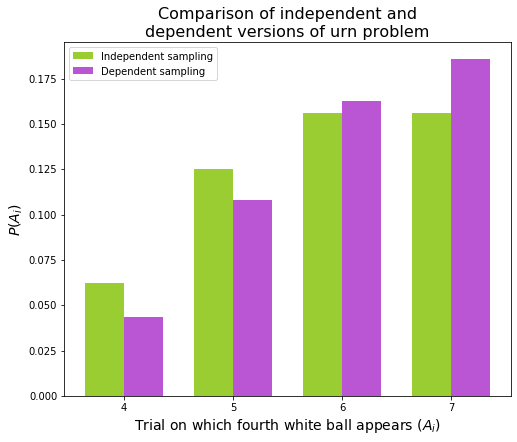My intuition says that the independent model should be a decent reflection of what happens in the World Series. While the relative skill of the teams may be different (white probably doesn’t have an exactly 50% chance of winning), it doesn’t seem to me that each team is drawing on a finite supply of wins or that their performance will deteriorate the more games they win.

On the other hand, perhaps there is an underdog phenomenon in which teams that are falling behind tend to exert themselves in hopes of a comeback victory.

On a third hand, perhaps losing teams tend to lose hope and give up after a few early losses.

If either of the previous two assertions are true, then we must discard the independent model. However, there are all sorts of dependent models possible, and the one we created above may be even worse than the independent model. In the end, neither model is suited to the real data that the textbook provides. Let’s add that to our graph, and this time we will double the probabilities obtained in the urn problem to account for the fact that four wins by either team ends the World Series:

In :
ws = np.array([21, 24, 23, 36])
# Divide by the sum to get the experimental probability
ws = ws / ws.sum()

In :
x=np.arange(4,8)
width=0.25
x1 = x - width
x2 = x + width

plt.figure(figsize=(8,6.5))
# Double the models since now we allow either team to win
plt.bar(x=x1,height=2*ways*psn,width=width,color='yellowgreen',label='Independent sampling model')
plt.bar(x=x,height=2*ways*ptn,width=width,color='mediumorchid',label='Dependent sampling model')
plt.bar(x=x2,height=ws,width=width,color='deepskyblue',label='Actual World Series lengths')

plt.xlabel(r'Length of World Series ($A_i$)',size=14)
plt.ylabel(r'$P(A_i)$',size=14)
plt.title('Comparison of independent and dependent\nversions of the World Series problem',size=16)
plt.xticks(x)
plt.legend()

Out:
<matplotlib.legend.Legend at 0x20c7ffd47b8>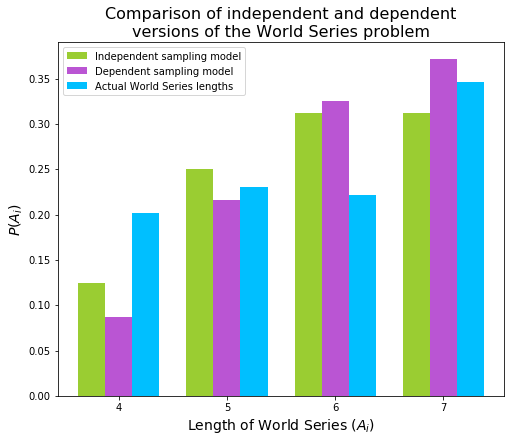As you can see, neither model accurately predicted the true distribution of World Series lengths. It is probably reasonable to reject the hypothesis that the outcomes of the games in the World Series are completely independent of one another.

## An optimization problem involving the gamma distribution¶

Reference: Robert V. Hogg et al., Probability and Statistical Inference 9e, section 3.2.

A bakery sells rolls in units of a dozen. The demand $X$ (in 1000 units) for rolls has a gamma distribution with parameters $\alpha=3$, $\theta = 0.5$, where $\theta$ is in units of days per 1000 units of rolls. It costs \$2 to make a unit that sells for \$5 on the first day when the rolls are fresh. Any leftover units are sold on the second day for \$1. How many units should be made to maximize the expected value of the profit? We can start by setting up the demand function, which is $$f(x) = \frac{x^{3 - 1}e^{-x/0.5}}{\Gamma(3)(0.5)^3} = 4x^2e^{-2x}$$ Python can construct this gamma function for us using scipy.stats.gamma.pdf. Let’s take a look at that. In : def demand(x): return gamma.pdf(x, 3, scale=0.5) x= np.linspace(0, 6,301) plt.figure(figsize=(10,8)) plt.title(r'Demand function (gamma distribution with$\alpha=3$,$\theta = 0.5$)',size=16) plt.xlabel(r'$x$', size=14) plt.ylabel(r'density', size=14) plt.plot(x,demand(x), color='maroon') plt.vlines(1.5,0,demand(1.5),ls='--',color='maroon',alpha=0.7, label=r'$\mu=1.5$') plt.legend()  Out: <matplotlib.legend.Legend at 0x20c002a89e8>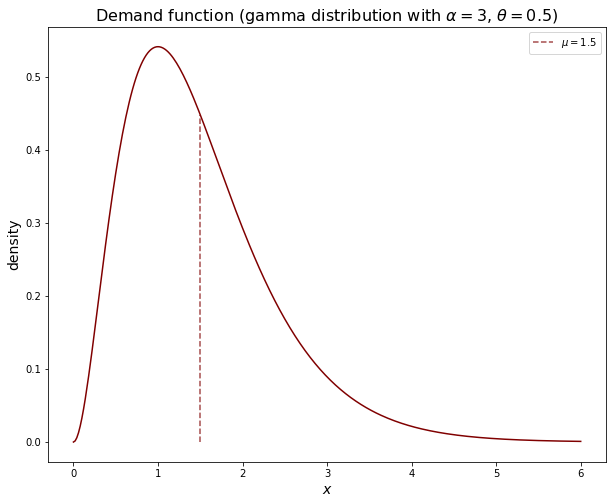Now, we need to set up our profit function. This is a function of$u$, which will denote the number of units we choose to make, and$x$. The function will have two pieces: If$u \lt x$, then the bakery will have leftovers and will need to sell them at reduced profit on the second day. If$u \geq x$, then we will simply sell all of the units at \$5 each. In either case, we will start by outlaying $2 per unit produced. Then $$C(u, x) = \begin{cases} -2u + 5u &= 3u, & u \lt x \\ -2u +5x + (u-x) &= 4x-u, & u \geq x \end{cases}$$ Since$x$is randomly determined, what we want to maximize is the expected value of$C(u,X). This we must break into two integrals because the profit function is piecewise. \begin{align}\mathbb{E}\left[C(u,X)\right] &= \int_{0}^{\infty}C(u,x) f(x)\,dx \\ & = \int_{0}^{u}(4x-u) 4x^2e^{-2x} \,dx + \int_{u}^{\inf}(3u) 4x^2e^{-2x} \,dx \\ \end{align} Breaking up the integrals further, we discover that the expected profit function is just made up of several gamma cdfs: \begin{align}\mathbb{E}\left[C(u,X)\right] &= 6\int_{0}^{u}\frac{16}{6}x^3e^{-2x} \,dx - u \int_{0}^{u} 4x^2e^{-2x} \,dx + 3u\int_{u}^{\inf} 4x^2e^{-2x} \,dx \\ &= 6 G(u) - u F(u) + 3u(1-F(u)) \\ &= 3u + 6 G(u) - 4u F(u)\end{align} whereG(u)$is the gamma cdf with$\alpha=4$,$\theta = 0.5$and$F(u)$is the gamma cdf with$\alpha=3$,$\theta = 0.5$. This will make our life a lot easier, since scipy has the gamma cdf built in. To solve algebraically, we’d have to apply serial integration by parts to integrate the gamma pdfs, and even that would leave us a messy polynomial whose roots we’d have to plug into the computer. We will define the profit function in Python as expected_profit. In : def expected_profit(u): return 3*u + 6*gamma.cdf(u, 4, scale=0.5) - 4*u*gamma.cdf(u, 3, scale=0.5)  Now that we have reduced our expected cost to a function of$u$, we need to find the maximum of this function. We know$u$will be positive, so we will ask scipy to start at$u=0$and move to the right. Scipy only does minimization, so we feed it the negative of our expected profit function. In : out = minimize(lambda u: - expected_profit(u), x0=0) profit = -out.fun best_u = out.x best_u, profit  Out: (1.9602010931382516, 3.3049588923017295) Looks like the optimal number of units to produce is 1960, corresponding to an expected profit of \$3305. Let’s plot this, and while we’re at it, we will correct the scale from showing the units in thousands.

In :
u= np.linspace(0,7,351)

plt.figure(figsize=(10,8))
plt.title(r'Expected profit', size=16)
plt.axhline(color='black',lw=1)
plt.plot(1000 * u, 1000 * expected_profit(u), color='royalblue')
plt.plot(1000 * best_u, 1000 * profit,'o', label='Maximum expected profit:\n1960 units, \\$3305',color='black') plt.xlabel(r'$u$', size=14) plt.ylabel('expected profit', size=14) plt.legend(loc='upper right')  Out: <matplotlib.legend.Legend at 0x20c00813710>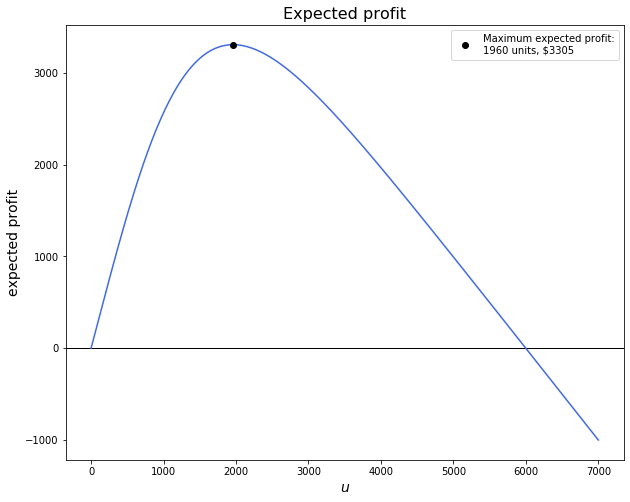This matches the answer given in the text. ## Derivation of the least-squares line of best fit¶ Reference: Robert V. Hogg et al., Probability and Statistical Inference 9e, section 4.2. Online, you can find many derivations of the least-squares fit for when you are given$X$and$Y$as points of data. The textbook asks us to prove this when$X$and$Y$are random variables distributed according to some pmf$f(x, y)$. The proof is essentially the same, but the symbols are different. Problem 5: Let$X$and$Y$be random variables with respective means$\mu_X$and$\mu_Y$, respective variances$\sigma_X^2$and$\sigma_Y^2$, and correlation coefficient$\rho$. Fit the line$y = a + bx$by the method of least squares to the probability distribution by minimizing the expectation$ K(a,b) = \mathbb{E}\left[(Y − a − bX)^2 \right]$with respect to$a$and$b. We have \begin{align} K(a,b) & = \mathbb{E}\left[(Y − a − bX)^2 \right] \\ & = \mathbb{E}\left(X^2 b^2 - 2 XYb + 2X ab + Y^2 - 2Ya + a^2\right) \\ & = b^2 (\sigma_X^2 + \mu_X^2) - 2b(\sigma_{XY} + \mu_X \mu_Y) + 2ab\mu_X + \sigma_Y^2 + \mu_Y^2 - 2a\mu_Y + a^2 \end{align} by expanding, distributing, then substituting\mathbb{E}(X^2) = \sigma_X^2 + \mu_X^2$,$\mathbb{E}(Y^2) = \sigma_Y^2 + \mu_Y^2$, and$\mathbb{E}(XY) = \sigma_{XY} + \mu_X \mu_Y$. Let’s set the first derivatives to zero and solve for$a$and$b. \begin{align} \frac{\partial K}{\partial a} = 0 & = 2b\mu_X - 2\mu_Y + 2a \\ & \implies \bbox[4px, border: 1px solid black]{ a = \mu_Y - b\mu_X } \\ \frac{\partial K}{\partial b} = 0 & = 2b(\sigma_X^2 + \mu_X^2) - 2 (\sigma_{XY} + \mu_X \mu_Y) + 2a \mu_X \\ & = 2b(\sigma_X^2 + \mu_X^2) - 2 (\sigma_{XY} + \mu_X \mu_Y) + 2(\mu_X \mu_Y - b \mu_X^2) \\ & = 2b\sigma_x^2 - 2\sigma_{XY} \\ & \implies \bbox[4px, border: 1px solid black]{ b = \frac{\sigma_{XY}}{\sigma_X^2} = \rho \frac{\sigma_Y}{\sigma_X} } \end{align} Then \begin{align} y & = a+bx \\ & = \mu_Y - b\mu_X + bx \\ & = \mu_Y + b(x - \mu_X) \\ & \bbox[4px, border: 1px solid black]{ = \mu_Y + \rho \frac{\sigma_Y}{\sigma_X} (x-\mu_X) } \end{align} This is the form we are familiar with. Now we just need to show that this is, in fact, a minimum rather than a maximum or saddle point. Taking second derivatives: \begin{align} \frac{\partial^2 K}{\partial a^2} & = 2 \\ \frac{\partial^2 K}{\partial b^2} & = 2 (\sigma_X^2 + \mu_X^2) \\ \frac{\partial^2 K}{\partial a \, \partial b} & = 2\mu_X \\ \end{align} In multiple variables, the second-derivative test uses the determinant of the Hessian matrix: \begin{align} \det \begin{bmatrix} \frac{\partial^2 K}{\partial a^2} & \frac{\partial^2 K}{\partial a \, \partial b} \\ \frac{\partial^2 K}{\partial b \, \partial a} & \frac{\partial^2 K}{\partial b^2} \\ \end{bmatrix} & = 2 \cdot 2 (\sigma_X^2 + \mu_X^2) - (2\mu_X)^2 \\ & = 4\sigma_X^2> 0 \\ \end{align} The determinant is positive, as are\frac{\partial^2 K}{\partial a^2}$and$\frac{\partial^2 K}{\partial b^2}$, so we have found a minimum. ## Plotting a discrete, bivariate probability distribution¶ References: Problem 11 references an interesting distribution: Choose a random integer$X$from the interval$[0, 4]$. Then choose a random integer$Y$from the interval$[0, x]$, where$x$is the observed value of$X$. Make assumptions about the marginal pmf$f_X (x)$and the conditional pmf$h(y | x)$and compute$P(X + Y > 4)$. By inspection, we see that $$f_X (x) = 1/5, \quad x= 0, 1, 2, 3, 4$$ Then, the number of choices for$Y$is restricted by$x$, so $$h(y|x) = \frac{1}{x+1}, \quad 0 \leq y \leq x, \quad x \text{ is one of } \{0, 1, 2, 3, 4\}$$ Since$ h(y|x) = \frac{f(x, y)}{f_X (x)} , we can work backwards to find the joint pmf: \begin{align} f(x, y) & = f_X (x) \cdot h(y|x) \\ & = \frac{1}{5}\cdot\frac{1}{x+1} \\ & = \frac{1}{5x+5}, \quad 0 \leq x \leq 4, \quad 0 \leq y \leq x \end{align} There are six points for whichx + y > 4$:$(3, 2),(3, 3),(4, 1),(4, 2),(4, 3),(4, 4)$. Since$f(x, y)$depends only on the value of$x$, the sum of$f(x,y)$over this space is$2/20 + 4/25 = 13/50$. Let’s plot$f(x,y)$. First we need all the points for which$ f(x, y) \neq 0$. In : h = np.array([[x, y] for x in range(5) for y in range(x+1)]) h  Out: array([[0, 0], [1, 0], [1, 1], [2, 0], [2, 1], [2, 2], [3, 0], [3, 1], [3, 2], [3, 3], [4, 0], [4, 1], [4, 2], [4, 3], [4, 4]]) Since the function is discrete and bound, one way to visualize it is by annotating each of the points. In : # Separate the x and y coords for easy plotting x, y = h.T # Compute the probabilities f(x,y) f = 1/ (5*x + 5) colors = plt.cm.rainbow(f.flatten()/float(f.max())) plt.figure(figsize=(8,7)) plt.title(r'$ f(x,y) = {1}/(5x+5), \quad 0 \leq x \leq 4, \quad 0 \leq y \leq x $', size=16) plt.scatter(x,y,color=colors,s=80,edgecolor="black") plt.xticks(np.arange(0,5)) plt.yticks(np.arange(0,5)) plt.xlim(-0.2,4.5) plt.xlabel('x', size=14) plt.ylabel('y', size=14) for i, z in enumerate(f): plt.annotate(np.round(z, decimals=3), xy=(x[i] + 0.1 ,y[i]), va='center')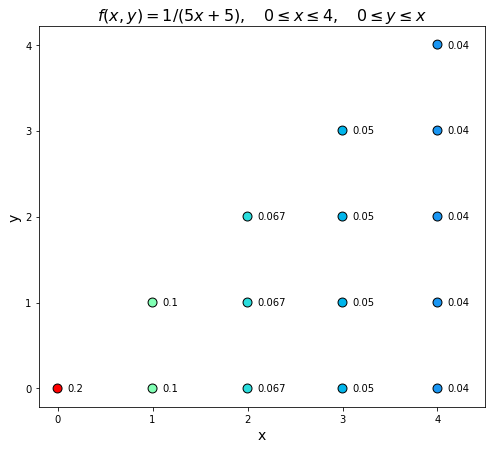Or, we can use a 3D bar chart. Matplotlib’s 3D plotting is still pretty buggy, so we have to tweak the viewport or it will fail to draw some faces. In : fig = plt.figure(figsize=(10,8)) ax = fig.gca(projection = '3d') ax.view_init(azim=-40, elev=28) ax.bar3d(x-0.5, y-0.5, np.zeros_like(f), dx = 1, dy = 1, dz = f,color=colors,edgecolor="black",lw=0.5,shade=True) ax.set_xlabel('x', size=14) ax.set_xlim(-.5, 4.5) ax.set_ylabel('y', size=14) ax.set_ylim(-.5, 4.5) ax.set_zlabel("probability", size=14,rotation=0) ax.set_zticks(np.arange(0,0.25,0.05)) ax.set_title(r'$ f(x,y) = {1}/(5x+5), \quad 0 \leq x \leq 4, \quad 0 \leq y \leq x $', size=16)  Out: Text(0.5, 0.92, '$ f(x,y) = {1}/(5x+5), \\quad 0 \\leq x \\leq 4, \\quad 0 \\leq y \\leq x ')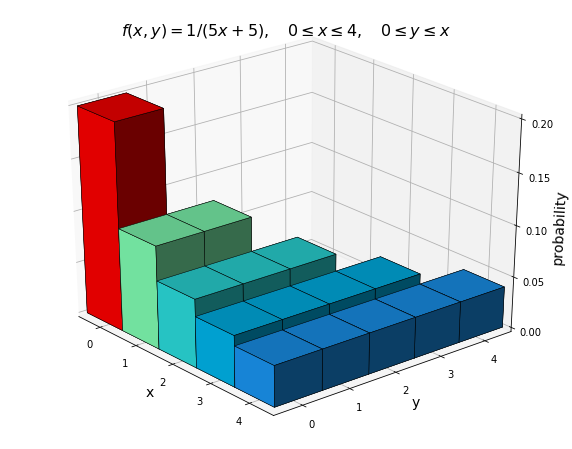## Plotting a bivariate normal pdf¶ Reference: Robert V. Hogg et al., Probability and Statistical Inference 9e, section 4.5. I reconstruct example 3, which has \begin{align} \mu_X & = 10 && \mu_Y = 12 \\ \sigma_X^2 & = 9 && \sigma_Y^2 = 16 \\ && \rho = 0.6 \\ \end{align} The conditional mean line is\mathbb{E}(Y | x) = 0.8x +4$. Then $$h(y|x) = \frac{1}{4\sqrt{2\pi}\sqrt{1-0.6^2}} \exp\left(- \frac{\left[y - \mathbb{E}\left(y|x\right) \right]^2}{2\cdot16\cdot(1-0.6^2)}\right)$$ In : def cond_mean(x): return 0.8*x + 4 def cond_pdf(x, y): return np.exp(- (y - cond_mean(x))**2 / (32*.64) ) / (4*np.sqrt(2*0.64*np.pi) ) $X$is also normal, so we have $$f_X(x) = N(10,9)$$ And $$f(x, y) = h(y|x) \cdot f_X(x)$$ In : def x_pdf(x): return norm.pdf(x,loc=10, scale=3) def joint_pdf(x, y): return x_pdf(x) * cond_pdf(x, y)  In the graph below, you can see the elliptical shape of the contours as well as the fact that the conditional mean line meets the contours at vertical tangents. I wonder about the statistical properties of a line of best fit that sits along the major axis of these ellipses instead. I believe this would be the line that minimizes the squared distance between$(X,Y)$and the nearest point to$(X,Y)$on the fit line. In : u = np.linspace(0,20,601) v = cond_mean(u) x, y = np.meshgrid(u, np.linspace(0,24,721)) z = joint_pdf(x,y) plt.figure(figsize=(8,9)) plt.title(r'Binormal pdf,$ \mu_X = 10, \mu_Y = 12, \sigma_X^2 = 9, \sigma_Y^2 = 16, \rho = 0.6 $', size=16) plt.contourf(x, y, z,cmap='magma') plt.plot(u,v, color="white") plt.xticks(np.arange(0,21,4)) plt.yticks(np.arange(0,25,4)) plt.xlabel('x', size=14) plt.ylabel('y', size=14)  Out: Text(0, 0.5, 'y')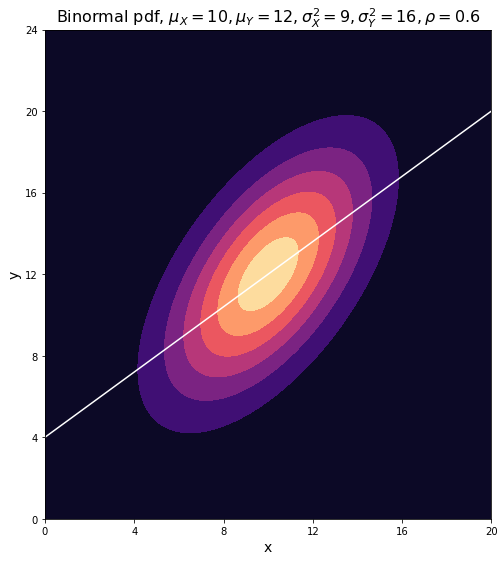In : #3D version of the same fig = plt.figure(figsize=(11,8)) ax = fig.gca(projection = '3d') ax.view_init(azim=-80, elev=50) ax.plot_wireframe(x, y, z, linewidth=1.5,edgecolor="black",alpha=0.7) plt.title(r'Binormal pdf,$ \mu_X = 10, \mu_Y = 12, \sigma_X^2 = 9, \sigma_Y^2 = 16, \rho = 0.6 $', size=16) ax.set_xticks(np.arange(0,21,4)) ax.set_yticks(np.arange(0,25,4)) ax.set_zticks(np.arange(0,0.017,0.005)) ax.set_xlabel('x', size=14) ax.set_ylabel('y', size=14) ax.set_zlabel('density', size=14)  Out: Text(0.5, 0, 'density')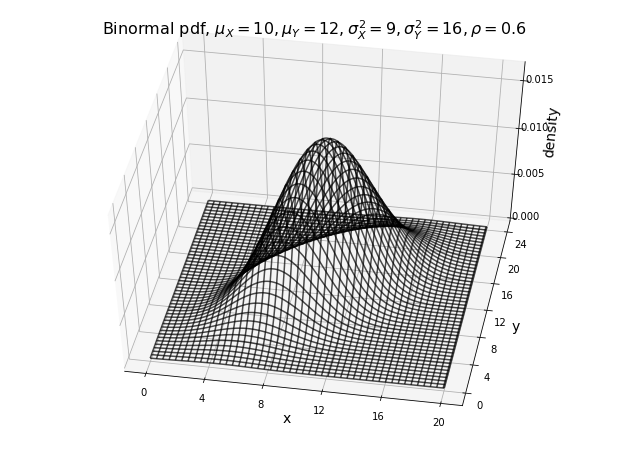## Preparation for the Central Limit Theorem¶ Reference: Robert V. Hogg et al., Probability and Statistical Inference 9e, section 5.4. Problem 13 reads, Let$X_1,X_2,\dots,X_8$be a random sample from a distribution having pmf$f(x) = (x + 1)/6, x = 0,1,2$. 1. Use Exercise 5.4-9 to find the pmf of$W_1 = X_1 + X_2$. 2. What is the pmf of$W_2 = X_3 + X_4$? 3. Now find the pmf of$W = W_1 + W_2 = X_1 + X_2 +X_3 + X_4$. 4. Find the pmf of$Y = X_1 + X_2 + \cdots + X_8$. 5. Construct probability histograms for$X_1$,$W_1$,$W$, and$Y$. Are these histograms skewed or symmetric? The result proven in 5.4-9 was the discrete convolution formula: The pmf of$W = X+Y$, where$X$and$Y$are independent random variables whose supports are subsets of the nonnegative integers, is $$h(w)= \sum_{x=0}^{w} f(x) g(w-x), \qquad w = 0, 1, 2, \dots$$ #### Part A¶$X_1$and$X_2$have the same pmf,$f(x)$. Note that the limits of the convolution summation do not account for places where$f(x) = 0$(namely, anywhere not on the space), so we need to include this condition when defining f(x) in Python. In : def f(x): if 0 <= x <= 2: return (x+1)/6 else: return 0 x1 = np.array([[i, f(i)] for i in range(3)]) x1  Out: array([[0. , 0.16666667], [1. , 0.33333333], [2. , 0.5 ]]) Then the pmf of$W_1$, which I call h(w), will perform the summation. In : def h(w): return sum( [f(x)*f(w-x) for x in range(0,w+1) ] )  By inspection, the support of$W_1$is$\{0, 1, 2, 3, 4\}$. Let’s get h(w) for those values and put it into an array, since in part E we will need to make a bar graph. In : a = np.array([[i, h(i)] for i in range(5)]) a  Out: array([[0. , 0.02777778], [1. , 0.11111111], [2. , 0.27777778], [3. , 0.33333333], [4. , 0.25 ]]) That is, $$h(w) = \begin{cases} 1&/\,36, & w = 0 \\ 4&/\,36, & w = 1 \\ 10&/\,36, & w = 2 \\ 12&/\,36, & w = 3 \\ 9&/\,36, & w = 4 \\ 0 & & \text{elsewhere} \\ \end{cases}$$ (The “elsewhere” case corresponds to places where$f(x) = 0$anyway, so we do not need to add cases to the definition of h(w).) #### Part B¶$X_3$and$X_4$have the same pmf as$X_1$and$X_2$, so the pmf of$W_2$will be the same:$h(w)$. #### Part C¶$W_1$and$W_2$themselves meet the criteria for the convolution formula, so we can apply it again, this time replacing$h(w) = \sum_{x=0}^{w} f(x) f(w-x)$with $$u(v) = \sum_{w=0}^{v} h(w) h(v-w)$$ The support will be$\{0,1, \dots, 8\}$. Making a similar array: In : def u(v): return sum( [h(w)*h(v-w) for w in range(0,v+1) ] ) c = np.array([[i, u(i)] for i in range(9)]) c  Out: array([[0.00000000e+00, 7.71604938e-04], [1.00000000e+00, 6.17283951e-03], [2.00000000e+00, 2.77777778e-02], [3.00000000e+00, 8.02469136e-02], [4.00000000e+00, 1.65123457e-01], [5.00000000e+00, 2.40740741e-01], [6.00000000e+00, 2.50000000e-01], [7.00000000e+00, 1.66666667e-01], [8.00000000e+00, 6.25000000e-02]]) This is $$u(v) = \begin{cases} 1 &/\, 36^2, & v = 0 \\ 8 &/\, 36^2, & v = 1 \\ 36 &/\, 36^2, & v = 2 \\ 104 &/\, 36^2, & v = 3 \\ 213 &/\, 36^2, & v = 4 \\ 311 &/\, 36^2, & v = 5 \\ 323 &/\, 36^2, & v = 6 \\ 216 &/\, 36^2, & v = 7 \\ 81 &/\, 36^2, & v = 8 \\ 0 & & \text{elsewhere}\\ \end{cases}$$ We might check that the values of$u(v)$actually sum to one. In : c[:,1].sum()  Out: 0.9999999999999999 Good enough. #### Part D¶ By the same logic, we have $$t(y) = \sum_{v=0}^{y} u(v) u(y-w), \qquad y = {0,1,\dots, 16}$$ In : def t(y): return sum( [u(v)*u(y-v) for v in range(0,y+1) ] ) d = np.array([[i, t(i)] for i in range(17)]) d  Out: array([[0.00000000e+00, 5.95374181e-07], [1.00000000e+00, 9.52598689e-06], [2.00000000e+00, 8.09708886e-05], [3.00000000e+00, 4.66773358e-04], [4.00000000e+00, 2.01712772e-03], [5.00000000e+00, 6.86823655e-03], [6.00000000e+00, 1.89710029e-02], [7.00000000e+00, 4.32194025e-02], [8.00000000e+00, 8.19461115e-02], [9.00000000e+00, 1.29658208e-01], [1.00000000e+01, 1.70739026e-01], [1.10000000e+01, 1.85442387e-01], [1.20000000e+01, 1.63387346e-01], [1.30000000e+01, 1.13425926e-01], [1.40000000e+01, 5.90277778e-02], [1.50000000e+01, 2.08333333e-02], [1.60000000e+01, 3.90625000e-03]]) Or $$t(y) = \begin{cases} 0 &/\,36^4, & y = 0 \\ 15 &/\,36^4, & y = 1 \\ 136&/\,36^4, & y = 2 \\ 783 &/\,36^4, & y = 3 \\ 3387 &/\,36^4, & y = 4 \\ 11535 &/\,36^4, & y = 5 \\ 31864 &/\,36^4, & y = 6 \\ 72591 &/\,36^4, & y = 7 \\ 137637 &/\,36^4, & y = 8 \\ 217775 &/\,36^4, & y = 9 \\ 286775 &/\,36^4, & y = 10 \\ 311471 &/\,36^4, & y = 11 \\ 274427 &/\,36^4, & y = 12 \\ 190511 &/\,36^4, & y = 13 \\ 99144 &/\,36^4, & y = 14 \\ 34992&/\,36^4, & y = 15 \\ 6561 &/\,36^4, & y = 16 \\ 0 & & \text{elsewhere}\\ \end{cases}$$ #### Part E¶ Now, we will make histograms showing the distributions of$X_1$,$W_1$,$W$, and$Y$, which I have stored in the variables x1, a, c, and d, respectively. In : distrs = [x1, a, c, d] titles = [r"$X_1\:(n=1)$", r"$W_1\:(n=2)$", r"$W\:(n=4)$", r"$Y\:(n=8)$"] colors = cm.get_cmap("Dark2")(np.linspace(0,.75,4)) fig, axs = plt.subplots(4, 1, sharex=True,figsize=(7, 14)) fig.subplots_adjust(hspace=0.3) fig.suptitle('Larger sample size increases symmetry', size=18, y=0.93) for i, dist in enumerate(distrs): axs[i].bar(*dist.T, color=colors[i], width=1) axs[i].set_title(titles[i],size=14) axs[i].set_xticks(np.arange(0,17)) axs[i].set_xticklabels(np.arange(0,17)) axs[i].tick_params(labelbottom=True)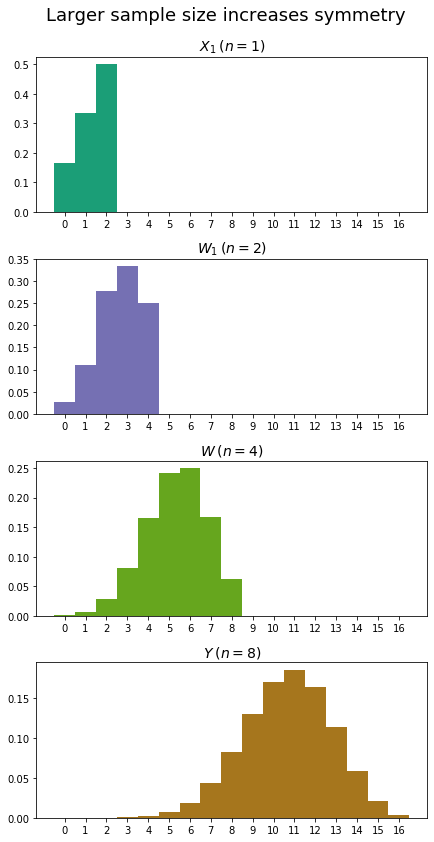As expected, the plot becomes more symmetrical as the sample size increases. ## Linear combination of independent geometric distributions¶ Reference: Robert V. Hogg et al., Probability and Statistical Inference 9e, section 5.4. Problem 15 reads, Given a fair four-sided die, let$Y$equal the number of rolls needed to observe each face at least once. 1. Argue that$Y = X_1 + X_2 + X_3 + X_4$, where$X_i$has a geometric distribution with$p_i = (5−i)/4$,$i = 1,2,3,4$, and$X_1$,$X_2$,$X_3$,$X_4$are independent. 2. Find the mean and variance of$Y$. 3. Find$\mathrm{P}(Y = y)$,$y = 4,5,6,7$. #### Part A¶ Assign$X_1$to whatever face appears first. Then$X_1$has a geometric distribution with$p=1$. That is,$\mathrm{P}(X_1 = 0) = 1$. Then, assign$X_2$to the first different face to appear. The chance of getting a different face is$3/4$, and$X_2$, the number of trials until it comes up, is geometric with$p=3/4$. Continuing in this manner, we have$X_3$with$p=2/4$and$X_4$with$p=1/4$, so each$X_i$is geometric with$p_i = (5−i)/4$. Then$Y = X_1 + X_2 + X_3 + X_4 . #### Part B¶ From our knowledge of the geometric distribution, we have \begin{align} \mu_{X_i} & = \frac{1}{p_i} \\ \sigma_{X_i}^2 & = \frac{1-p}{p^2} \\ \end{align}Y$is a linear combination of the$X_i$s with each$a_i = 1, so \begin{align} \mu_Y & = \sum_{i=1}^4 \mu_{X_i} = \frac{4}{4} + \frac{4}{3}+ \frac{4}{2}+ \frac{4}{1} = \bbox[4px, border: 1px solid black]{\frac{25}{3}} \\ \sigma_Y^2 & = \sum_{i=1}^4 \sigma_{X_i}^2 = 0 + \frac{1}{4}\left(\frac{4}{3}\right)^2 + \frac{2}{4}\left(\frac{4}{2}\right)^2 + \frac{3}{4}\left(\frac{4}{1}\right)^2 = \bbox[4px, border: 1px solid black]{\frac{130}{9} } \\ \end{align} #### Part C¶ The mgf of the geometric distribution is $$M_{X_i}(t) = \frac{p_i e^t}{1- (1-p_i)e^t}$$Y$is a linear combination of the$X_is, so \begin{align} M_Y (t) & = \prod_{i=1}^4 M_{X_i} \\ & = \frac{e^t}{1} \cdot \frac{\frac{3}{4} e^t}{1- \left(1-\frac{3}{4}\right)e^t}\cdot\frac{\frac{1}{2} e^t}{1- \left(1-\frac{1}{2}\right)e^t} \cdot\frac{\frac{1}{4} e^t}{1- \left(1-\frac{1}{4}\right)e^t} \\ & = \frac{3}{32} \cdot \frac{e^{4t}}{(1- \frac{3}{4}e^t)(1- \frac{1}{2}e^t)(1- \frac{1}{4}e^t)} \\ \end{align} We can double-check (or perform) this simplification using sympy: In : from sympy.abc import p, t Mx = p*sympy.exp(t) / (1- (1-p)*sympy.exp(t)) My = np.prod([Mx.subs(p,i) for i in [1, .75, .5, .25 ]]) My.nsimplify()  Out:\displaystyle \frac{3 e^{4 t}}{32 \left(1 - \frac{3 e^{t}}{4}\right) \left(1 - \frac{e^{t}}{2}\right) \left(1 - \frac{e^{t}}{4}\right)}$Arranged like this, the mgf won’t help us evaluate the pmf$f(y)$, but we can use Taylor series to get it into a legible form. From$\frac{1}{1-az} = 1 + az + (az)^2 + (az)^3 + \dots, we have \begin{align} M_Y (t) = \frac{3}{32} e^{4t} & \left[1 + \left(\frac{3}{4}\right) e^t + \left(\frac{3}{4}\right)^2 e^{2t} + \left(\frac{3}{4}\right)^3 e^{3t} + \cdots \right] \\ \times & \left[1 + \left(\frac{1}{2}\right) e^t + \left(\frac{1}{2}\right)^2 e^{2t} + \left(\frac{1}{2}\right)^3 e^{3t} + \cdots \right] \\ \times & \left[1 + \left(\frac{1}{4}\right) e^t + \left(\frac{1}{4}\right)^2 e^{2t} + \left(\frac{1}{4}\right)^3 e^{3t} + \cdots \right] \\ \end{align} fort \lt \ln(4/3)$. The problem only asks us to evaluate the pmf of$y$up to$f(7)$, which will correspond in the mgf to the coefficient of$e^{7t}$. Since we have$e^{4t}$at the front, we won’t need any further terms than these to answer the question. All we need to do is multiply out the expression as shown above. We can do this using sympy. In : # First four terms of each Taylor series one = sum([0.75**n *sympy.exp(n*t) for n in range(4)]) two = sum([0.5**n *sympy.exp(n*t) for n in range(4)]) three = sum([0.25**n *sympy.exp(n*t) for n in range(4)]) My2 = (3/32) * sympy.exp(4*t) *one*two*three My2 = My2.expand() My2  Out:$\displaystyle 7.72476196289063 \cdot 10^{-5} e^{13 t} + 0.000566482543945313 e^{12 t} + 0.00291824340820313 e^{11 t} + 0.0131607055664063 e^{10 t} + 0.03460693359375 e^{9 t} + 0.0743408203125 e^{8 t} + 0.1318359375 e^{7 t} + 0.146484375 e^{6 t} + 0.140625 e^{5 t} + 0.09375 e^{4 t}$Messy, but we can use sympy to pull out the coefficients and the$y$-values. In : y = My2.as_coefficients_dict().keys() # Log out the exp, divide out t y = [(sympy.log(i) / t).expand(force=True) for i in y] f = list(My2.as_coefficients_dict().values()) pmf = np.stack([y,f]).T pmf = pmf[pmf[:,0].argsort()] pmf  Out: array([[4, 0.0937500000000000], [5, 0.140625000000000], [6, 0.146484375000000], [7, 0.131835937500000], [8, 0.0743408203125000], [9, 0.0346069335937500], [10, 0.0131607055664063], [11, 0.00291824340820313], [12, 0.000566482543945313], [13, 7.72476196289063e-5]], dtype=object) The values for$y>7are inaccurate because we didn’t include all the relevant terms from the Taylor expansion. But we have our answer. In : pmf[0:4,1] * 1024  Out: array([96.0000000000000, 144.000000000000, 150.000000000000, 135.000000000000], dtype=object) That is, \begin{align} f(4) & = 96 & /\:1024 \\ f(5) & = 144 & / \:1024 \\ f(6) & = 150 & / \:1024 \\ f(7) & = 135 & / \:1024 \\\end{align} Let’s make a histogram off(y)$. We will need more terms from the Taylor series. In : r = 20 one = sum([0.75**n *sympy.exp(n*t) for n in range(r)]) two = sum([0.5**n *sympy.exp(n*t) for n in range(r)]) three = sum([0.25**n *sympy.exp(n*t) for n in range(r)]) My3 = (3/32) * sympy.exp(4*t) *one*two*three My3 = My3.expand() y = My3.as_coefficients_dict().keys() y = [(sympy.log(i) / t).expand(force=True) for i in y] f = list(My3.as_coefficients_dict().values()) pmf = np.stack([y,f]).T pmf = pmf[pmf[:,0].argsort()] pmf = pmf[:r] # Only the first r values are accurate  In : plt.figure(figsize=(9,7)) colors = cm.get_cmap("winter")(0.8- 0.6*(pmf[:,1] / pmf[:,1].max()).astype(float)) plt.bar(*pmf.T,color=colors, width=1) plt.xlabel(r'$ y = x_1 + x_2 + x_3 + x_4$',size=14) plt.xticks(np.arange(r+4)) plt.xlim(-0.5, r+3.5) plt.ylabel(r'$ f(y)$',size=14) plt.title('Linear combination of independent geometric distributions',size=16)  Out: Text(0.5, 1.0, 'Linear combination of independent geometric distributions')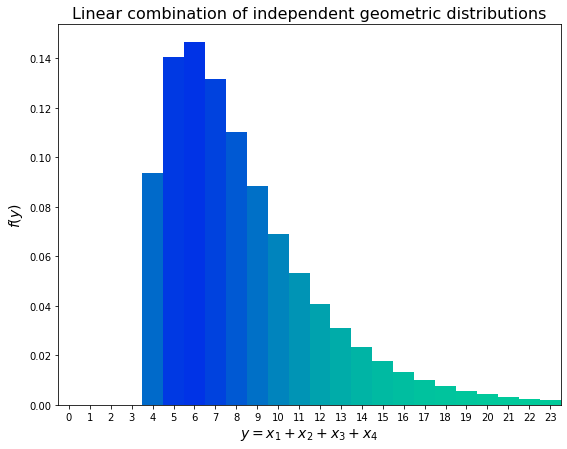## Reverse Chebyshev¶ Reference: Robert V. Hogg et al., Probability and Statistical Inference 9e, section 5.8. Problem 7 reads, Suppose that$W$is a continuous random variable with mean 0 and a symmetric pdf$f(w)$and cdf$F(w)$, but for which the variance is not specified (and may not exist). Suppose further that$W$is such that $$\mathrm{P}\left(\left|W − 0\right| \lt k\right) = 1 − \frac{1}{k^2}$$ for$k \geq 1$. (Note that this equality would be equivalent to the equality in Chebyshev’s inequality if the variance of$W$were equal to 1.) Then the cdf satisfies $$F(w) − F(−w) = 1 − \frac{1}{w^2}, \qquad w \geq 1.$$ Also, the symmetry assumption implies that $$F(−w) = 1 − F(w).$$ 1. Show that the pdf of$W$is $$f(w) = \begin{cases}\frac{1}{\left|w\right|^3}, & \left|w\right| \gt 1 \\ 0, & \left|w\right| \leq 1.\end{cases}$$ 2. Find the mean and the variance of$W$and interpret your results. 3. Graph the cdf of$W$. #### Part A¶ For$w \geq 1$, we have $$F(w) - F(-w) = F(w) - \left[1-F(w)\right] = 2F(w) -1 = 1 − \frac{1}{w^2} \\ \implies F(w) = 1 − \frac{1}{2w^2} \\ \implies \bbox[4px, border: 1px solid black]{ f(w) = \frac{1}{w^3}, \qquad w \geq 1 }$$ For negative$w, we apply the symmetry assumption: F(-w) = 1- F(w) \\ \begin{align}\implies F(w) & = 1 - F(\left|w\right|) \\ & = 1 - ( 1 - \frac{1}{2\left|w\right|^2}) \\ & = \frac{1}{2w^2}\end{align} \\ \implies \bbox[4px, border: 1px solid black]{f(w) = -\frac{1}{w^3}, \qquad w \leq -1} Notice thatF(-1) = F(1) = 1/2$, meaning that no probability is gained on$ -1 \lt w \lt 1$. This implies $$\bbox[4px, border: 1px solid black]{f(w) = 0, \qquad -1 \lt w \lt 1 \quad}$$ Put the three boxed portions together to get $$\bbox[4px, border: 1px solid black]{ f(w) = \begin{cases}\frac{1}{\left|w\right|^3}, & \left|w\right| \geq 1 \\ 0, & \left|w\right| \lt 1\end{cases} }$$ (I don’t know why the textbook swaps the inequalities for$\left|w\right|= 1$, but these are zero-probability events anyhow.) #### Part B¶ Note that we do not get$\mu_W = 0$for free from the symmetry assumption; a distribution can be symmetric with undefined$\mu. Here is the mean: \begin{align} \mathbb{E}\left(W\right) & = \int_{\infty}^{\infty} w \cdot f(w) \, dw \\ & = \int_{1}^{\infty} w^{-2} \,dw + \int_{-\infty}^{-1} - w^{-2} \, dw \\ & = \left[-\frac{1}{w}\right]_{1}^{\infty} + \left[\frac{1}{w}\right]_{-\infty}^{-1} \\ & = 1 - 1 = 0 & \implies \bbox[4px, border: 1px solid black]{ \mu_W = 0 } \end{align} And the standard deviation: \begin{align} \mathbb{E}\left[(W - \mu_W)^2\right] & = \int_{\infty}^{\infty} (w-0)^2 \cdot f(w) \,dw \\ & = \int_{1}^{\infty} \frac{1}{w} \,dw + \int_{-\infty}^{-1} \frac{1}{w} \,dw \\ & = \bigl[\ln\left|w\right|\bigr]_{1}^{\infty} + \bigl[\ln\left|w\right|\bigr]_{-\infty}^{-1} \\ & = \infty - \infty & \implies \bbox[4px, border: 1px solid black]{ \sigma_W^2 \text{ undefined} }\end{align} \\ #### Part C¶ Graphing the cdf. In : def cdf(w): if w <= -1: return 0.5 * w**-2 if -1 < w < 1: return 0.5 if w >= 1: return 1- 0.5 * w**-2 cdf = np.vectorize(cdf) t = np.linspace(-11, 11, 221) plt.figure(figsize=(10,8)) plt.title(r'cdf off(w) = \{\left|w\right|^{-3}, \quad \left|w\right| \geq 1; 0 \mathrm{\ elsewhere}\}$') plt.plot(t,cdf(t), color='forestgreen') plt.xlabel(r'$w$', size=14) plt.xlim(-11,11) plt.xticks(np.arange(-10,11, 2)) plt.ylim(0,1) plt.yticks(np.arange(0,1.01,.1)) plt.ylabel(r'$F(w)$', size=14)  Out: Text(0, 0.5, '$F(w)$')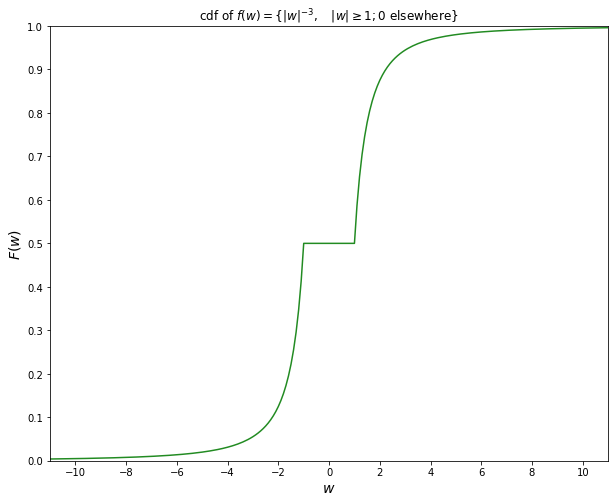## q–q plot Reference: Robert V. Hogg et al., Probability and Statistical Inference 9e, section 6.3. Problem 13 reads, Some measurements (in mm) were made on specimens of the spider Sosippus floridanus, which is native to Florida. Here are the lengths of nine female spiders and nine male spiders. [data below] 1. Construct a q–q plot of the female spider lengths. Do they appear to be normally distributed? 2. Construct a q–q plot of the male spider lengths. Do they appear to be normally distributed? In : females = np.array('11.06 13.87 12.93 15.08 17.82 14.14 12.26 17.82 20.17'.split()) males = np.array('12.26 11.66 12.53 13.00 11.79 12.46 10.65 10.39 12.26'.split()) females = np.vectorize(float)(females) males = np.vectorize(float)(males) females.sort() males.sort() pcs = np.linspace(0, 1, len(females)+2)[1:-1] npcs = norm.ppf(pcs) plt.figure(figsize=(8,8)) plt.scatter(females, npcs, color="blue", label="females") plt.scatter(males, npcs, color="crimson", label="males") plt.title(r'q–q plot of spider length', size=18) plt.ylabel(r'$N(0,1)$quantiles', size=14) plt.xlabel('length (mm)', size=14) plt.legend()  Out: <matplotlib.legend.Legend at 0x1ba175793c8>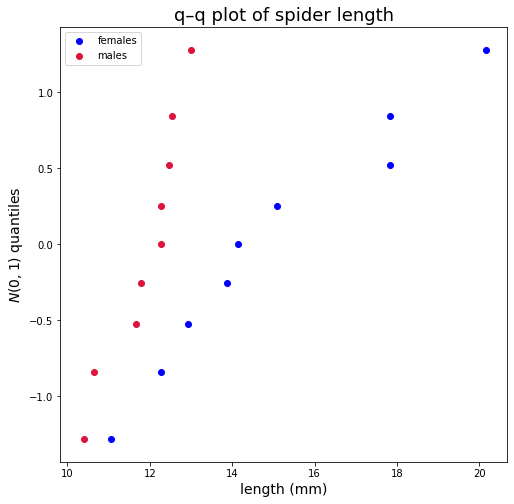Both sets of points follow roughly straight lines, suggesting that the data are normally distributed. ## Maximum likelihood estimation¶ Reference: Robert V. Hogg et al., Probability and Statistical Inference 9e, section 6.4. Problem 19 reads, Let the independent normal random variables$Y_1,Y_2 ,\dots,Y_n$have the respective distributions$N(\mu,\gamma^2 x_i^2), i = 1, 2, \dots ,n$, where$x_1,x_2 ,\dots, x_n$are known but not all the same and no one of which is equal to zero. Find the maximum likelihood estimators for$\mu$and$\gamma^2$. It’s not stated in the problem, but clear from the solution given that we also have a sample of size$ncomposed of one sample from each distribution, and we know which comes from where—not realistic, but theoretically interesting. For our likelihood function, we have \begin{align} L(\mu, \gamma) &= \prod_{i=1}^n \frac{1}{\sqrt{ 2\pi\gamma^2x_i^2}}\exp\left[-\frac{\left( y_i - \mu \right)^2}{2\gamma^2 x_i^2}\right] \\ &= \left(\frac{1}{\sqrt{ 2\pi\gamma^2}}\right)^n \frac{1}{\prod x_i}\exp\left[-\sum \frac{\left( y_i - \mu \right)^2}{2\gamma^2 x_i^2}\right] \\ \end{align} Our goal is to maximize this, so we can take the logarithm without affecting the location of the maximum: \begin{align} \ln\left[L(\mu, \gamma)\right] &= -\frac{n}{2}\ln(2\pi) - n\ln\gamma - \sum\ln(x_i) -\sum \frac{\left( y_i - \mu \right)^2}{2\gamma^2x_i^2} & \\ \frac{\partial}{\partial \mu} \ln\left[L(\mu, \gamma)\right] &= \sum \frac{\left( y_i - \mu \right)}{\gamma^2 x_i^2} = 0 & \\ &= \mu \sum \frac{1}{x_i^2} + \sum \frac{y_i}{x_i^2} & \implies \bbox[4px, border: 1px solid black]{ \hat\mu = \frac{\sum y_i \big/ x_i^2}{\sum 1 \big/ x_i^2} }\\ \frac{\partial}{\partial \gamma} \ln\left[L(\mu, \gamma)\right] &= -\frac{n}{\gamma} + \frac{1}{\gamma^3} \sum \frac{\left( y_i - \mu \right)^2}{x_i^2} = 0 \\ &= -n\gamma^2 + \sum \frac{\left( y_i - \mu \right)^2}{x_i^2} & \implies \bbox[4px, border: 1px solid black]{ \widehat{\gamma^2} = \frac{1}{n}\sum\frac{\left(y_i - \hat\mu\right)^2}{x_i^2} } \end{align} ## Basu’s theorem Reference: Robert V. Hogg et al., Probability and Statistical Inference 9e, section 6.7. Problem 9 reads, LetX_1 ,X_2 , \dots ,X_n$be a random sample from$N(\theta_1 ,\theta_2)$. Show that the sufficient statistics$Y_1 = \bar X$and$Y_2 = S^2$are independent of the statistic $$Z = \sum_{i=1}^{n−1} \frac{\left(X_{i+1} − X_i\right)^2}{S^2}$$ because Z has a distribution that is free of$\theta_1$and$\theta_2$. The mgf of$Zis \begin{align} \mathbb{E}(e^{tZ}) = \int_{-\infty}^{\infty} \int_{-\infty}^{\infty} \dotsi \int_{-\infty}^{\infty} {\exp\left[ t \sum_{i=1}^{n−1} \frac{\left(x_{i+1} − x_i\right)^2}{S^2}\right]} \frac{1}{\left(\sqrt{2 \pi \theta_2}\right)^n} {\exp \left[-\frac{\sum_{i=1}^{n} \left(x_{i} − \theta_1 \right)^2}{2\theta_2}\right]} {dx_1 dx_2 \dots dx_n} \end{align} As the text suggests, make the substitutionw_i = \frac{(x_i − \theta_1 )}{ \sqrt{\theta_2}}$. We also know$S^2 = \frac{1}{n-1}\sum_{i=1}^{n} (x_{i} - \theta_1)^2$. Then, working with the expression for$e^{tZ}$(the first$\expin the integral), we have \begin{align} \exp\left[ t \sum_{i=1}^{n−1} \frac{\left(x_{i+1} − x_i\right)^2}{S^2}\right] & = \exp\left[ t \frac{ \sum_{i=1}^{n−1} \bigl( (x_{i+1} - \theta_1)+ (\theta_1 − x_i)\bigr)^2}{\frac{1}{n-1}\sum_{i=1}^{n} (x_{i} - \theta_1)^2}\right] \\ & = \exp\left[ t \frac{\sum_{i=1}^{n−1} \left( \sqrt{\theta_2} w_{i+1} + \sqrt{\theta_2}w_i\right )^2}{\frac{1}{n-1}\sum_{i=1}^{n} ( \sqrt{\theta_2} w_i)^2} \right] \\ & = {\exp\left[ t (n-1) \frac{\sum_{i=1}^{n−1} \left( w_{i+1} + w_i\right )^2}{\sum_{i=1}^{n} (w_i)^2} \right]} \end{align} The other exponential expression is \begin{align} \exp \left[-\frac{\sum_{i=1}^{n} \left(x_{i} − \theta_1 \right)^2}{2\theta_2}\right] & = \exp \left[-\frac{\sum_{i=1}^{n} \left(\sqrt{\theta_2} w_i\right)^2}{2\theta_2}\right] \\ & = {\exp \left[-\frac{1}{2} \sum_{i=1}^{n} w_i^2\right]} \end{align} Finally, fromx_i = \sqrt{\theta_2} w_i + \theta_1$, we have$dx_i = \sqrt{\theta_2} dw_i$, so$dx_1 dx_2 \dots dx_n = {\left(\sqrt{\theta_2}\right)^n dw_1 dw_2 \dots dw_n}$.$\left(\sqrt{\theta_2}\right)^ncancels with the one in the denominator, and the final expression for the mgf is \begin{align} \mathbb{E}(e^{tZ}) = \int_{-\infty}^{\infty} \int_{-\infty}^{\infty} \dotsi \int_{-\infty}^{\infty} {\exp\left[ t (n-1) \frac{\sum_{i=1}^{n−1} \left( w_{i+1} + w_i\right )^2}{\sum_{i=1}^{n} (w_i)^2} \right]} \frac{1}{\left(\sqrt{2 \pi}\right)^n} {\exp \left[-\frac{1}{2} \sum_{i=1}^{n} w_i^2\right]} {dw_1 dw_2 \dots dw_n} \end{align} which is free of both parameters and therefore, by Basu’s theorem, independent ofY_1$and$Y_2$. References: • Robert V. Hogg et al., Probability and Statistical Inference 9e, section 7.6. • Gilbert Strang, Linear Algebra and Its Applications 4e, section 3.3. Problem 3 reads, For the data given in Exercise 6.5-3 [data below], with the usual assumptions, 1. Find a 95% confidence interval for$\mu(x)$when$x =$68, 75, and 82. 2. Find a 95% prediction interval for$Y$when$x =\$ 68, 75, and 82.

I created some simple Python functions useful in answering this sort of problem.

In :
from scipy.stats import t

def beta(x, y):
return y @ (x- x.mean()) / sum((x - x.mean())**2)

def line(x, y):
def mu(z):
return y.mean() + beta(x, y) * (z - x.mean())
return mu

def sigma2(x, y):
mu = line(x,y)
residuals = y - mu(x)
return sum(residuals**2) / len(y)

def c(x, y):
n = len(y)
def f(z):
return np.sqrt( n*sigma2(x,y) / (n-2)) * np.sqrt( 1/n + (z - x.mean())**2/sum((x - x.mean())**2))
return f

def d(x, y):
n = len(y)
def f(z):
return np.sqrt( n*sigma2(x,y) / (n-2)) * np.sqrt(1+ 1/n + (z - x.mean())**2/sum((x - x.mean())**2))
return f

In :
x, y = np.vectorize(int)(np.array('70 87 74 79 80 88 84 98 80 96 67 73 70 83 64 79 74 91 82 94'.split())).reshape(10,2).T
pred = line(x, y)

pt_a = []
for i in [68, 75, 82]:
pt_a.append(( pred(i) - c(x,y)(i) * t.isf(.025, len(y)-2), pred(i) + c(x,y)(i) * t.isf(.025, len(y)-2) ))
pt_a

Out:
[(75.28277625314745, 85.11336367447619),
(83.83843759007924, 90.77724395394971),
(89.10712853220839, 99.72809462822586)]
In :
pt_b = []
for i in [68, 75, 82]:
pt_b.append(( pred(i) - d(x,y)(i) * t.isf(.025, len(y)-2), pred(i) + d(x,y)(i) * t.isf(.025, len(y)-2)) )
pt_b

Out:
[(68.20615316762974, 92.1899867599939),
(75.83253186012546, 98.78314968390349),
(82.258368742456, 106.57685441797825)]

These match the answers in the text.

In :
def CI(i):
return ( pred(i) - c(x,y)(i) * t.isf(.025, len(y)-2), pred(i) + c(x,y)(i) * t.isf(.025, len(y)-2) )
def PI(i):
return ( pred(i) - d(x,y)(i) * t.isf(.025, len(y)-2), pred(i) + d(x,y)(i) * t.isf(.025, len(y)-2) )

x_plot = np.arange(62, 87)
CI_lower, CI_higher = CI(x_plot)
PI_lower, PI_higher = PI(x_plot)

plt.figure(figsize = (9, 9))
plt.fill_between(x_plot,PI_lower, PI_higher, color='lightsteelblue', label = '95% prediction interval')
plt.fill_between(x_plot,CI_lower, CI_higher, color='lightskyblue', label = '95% confidence interval')
plt.scatter(x,y, color='black')
plt.plot(x_plot, pred(x_plot), color = 'crimson', label = 'linear fit')

for i in [CI_lower, CI_higher, PI_lower, PI_higher]:
plt.plot(x_plot, i, color = 'black', lw=0.5)

plt.title('Simple linear regression', size=16)
plt.xlim(62, 86)
plt.legend()

Out:
<matplotlib.legend.Legend at 0x1b6c34dde80>